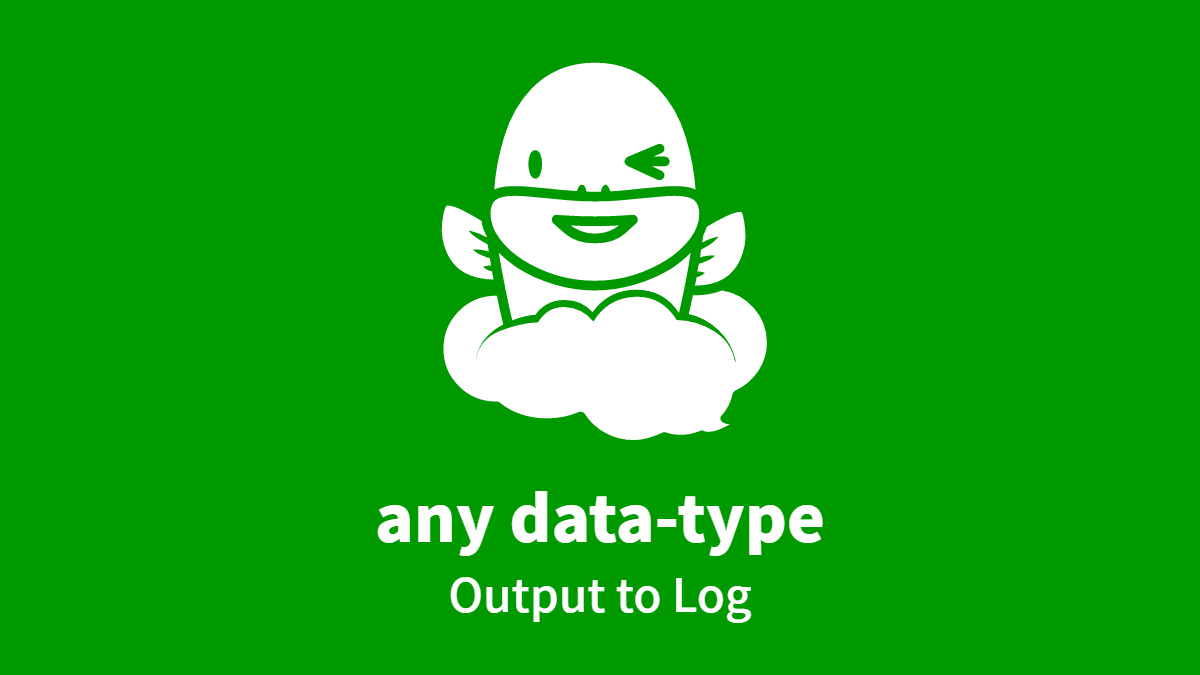any data-type, Output to Log
Outputs business data to the Auto-Processing Log. Administrators of the Workflow App can verify the data that was stored when the Process reached this step.
Configs
• A: Select DATA whose stored data will be written to log *
Script (click to open)
``````// GraalJS Script (engine type: 2)
/*
NOTES
- For example, an overwrite is expected in a downstream step.
- If no data is stored, this automated step will be abended with an error.
- Files type outputs "file name, file size, Content-Type" for all.
- Guide-Panel, Discussion, Table type not supported.
- In case Table type, consider "Update Data" or "Converter".
NOTE-ja
- 下流工程での上書きが想定されるような業務プロセスにおいて利用されます。
- データが何も格納されていない場合、この自動処理工程はエラー終了します。
- ファイル群型は、全てについて "ファイル名・ファイルサイズ・Content-Type" を出力します。
- ガイドパネル型、掲示板ディスカッション型、テーブル型は選択できません。
- テーブル型は「データ更新」や「コンバータ」の併用を検討します。
- タイトルは「データ更新」の併用を検討します。
*/

//////// START "main()" /////////////////////////////////////////////////////////////////
main();
function main(){

//// == Config Retrieving / 工程コンフィグの参照 ==
const multiPocketA = configs.getObject( "SelectConfA" ); // required

//// == Data Retrieving / ワークフローデータの参照 ==
if( engine.findData( multiPocketA ) === null ){
throw new Error( "\n AutomatedTask NullError:" +
" Nothing is stored in the specified variable (Data Definition) \n" );
}

//// == Calculating / 演算 ==
let strOutput = "";
if( multiPocketA.matchDataType("STRING") ){
multiPocketA.getName() + "'" );
strOutput = engine.findData( multiPocketA ) + "";
engine.log( strOutput );

}else if( multiPocketA.matchDataType("DECIMAL") ){
multiPocketA.getName() + "'" );
strOutput = engine.findData( multiPocketA ) + "";
engine.log( strOutput );

}else if( multiPocketA.matchDataType("DATE") ){
multiPocketA.getName() + "'" );
strOutput = engine.findData( multiPocketA ).toString() + "";
engine.log( strOutput );

}else if( multiPocketA.matchDataType("DATETIME") ){
multiPocketA.getName() + "'" );
strOutput = engine.findData( multiPocketA ).toString() + "";
engine.log( strOutput );

}else if( multiPocketA.matchDataType("SELECT") ){
multiPocketA.getName() + "'" );
let numSelected = engine.findData( multiPocketA ).size() - 0;
for ( let i = 0; i < numSelected; i++ ){
strOutput += "'";
strOutput += engine.findData( multiPocketA ).get(i).getValue();
strOutput += "': '";
strOutput += engine.findData( multiPocketA ).get(i).getDisplay();
strOutput += "'\n";
}
engine.log( strOutput );

}else if( multiPocketA.matchDataType("QUSER") ){
multiPocketA.getName() + "'" );
strOutput += "'";
strOutput += engine.findData( multiPocketA ).getId();
strOutput += "': '";
strOutput += engine.findData( multiPocketA ).getName();
strOutput += " <";
strOutput += engine.findData( multiPocketA ).getEmail();
strOutput += ">'";
engine.log( strOutput );

}else if( multiPocketA.matchDataType("QGROUP") ){
multiPocketA.getName() + "'" );
strOutput += "'";
strOutput += engine.findData( multiPocketA ).getId();
strOutput += "': '";
strOutput += engine.findData( multiPocketA ).getName();
if( engine.findData( multiPocketA ).getEmail() + "" === "" ){
strOutput += " <";
strOutput += engine.findData( multiPocketA ).getEmail();
strOutput += ">";
}
strOutput += "'";
engine.log( strOutput );

}else if( multiPocketA.matchDataType("FILE") ){
multiPocketA.getName() + "'" );
let numFiles = engine.findData( multiPocketA ).size() - 0;
for ( let i = 0; i < numFiles; i++ ){
strOutput += "'";
strOutput += engine.findData( multiPocketA ).get(i).getName();
strOutput += "': ";
strOutput += engine.findData( multiPocketA ).get(i).getContentType();
strOutput += " ";
strOutput += engine.findData( multiPocketA ).get(i).getLength();
strOutput += " bytes\n";
}
engine.log( strOutput );

}else{ // Unknown
throw new Error( "\n AutomatedTask CriticalError:" +
" DataType Unmatched \n" );
}

//// == Data Updating / ワークフローデータへの代入 ==
// (nothing)

} //////// END "main()" /////////////////////////////////////////////////////////////////

/*
APPENDIX
- Output Log sample1
- Suzuki
- Output Log sample2
- 12-31
- Output Log sample3
- 'Corp-Num-Web-API-Organizations-Search-ID-with-Name-en.png': image/png 56836 bytes
- 'Corp-Num-Web-API-Organizations-Search-Name-with-ID-en.png': image/png 56809 bytes
- Error Log sample
- AutomatedTask NullError: Nothing is stored in the specified variable (Data Definition)
*/

``````

The Addon-import feature is available with Professional edition.

### Appendix

• Output Log sample1
• Suzuki
• Output Log sample2